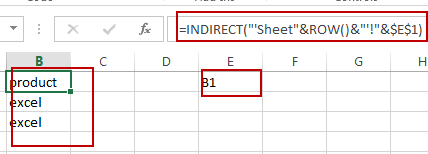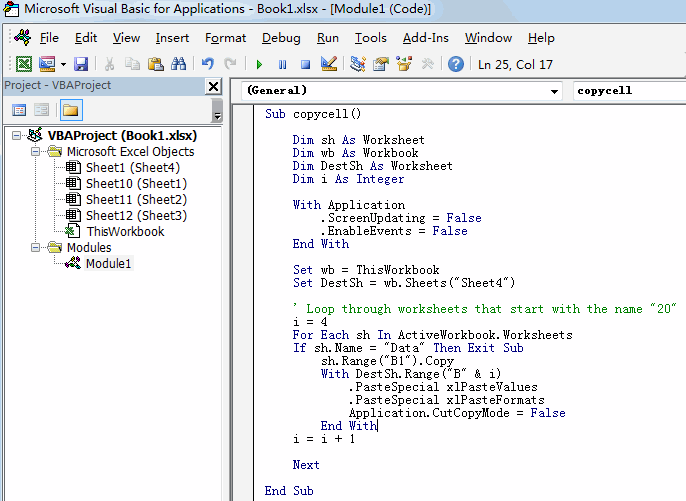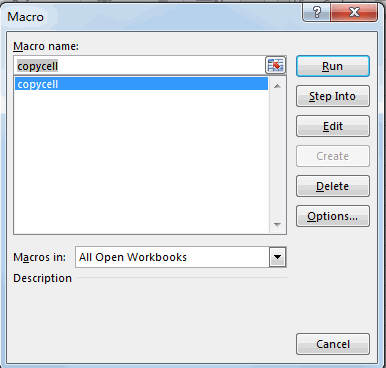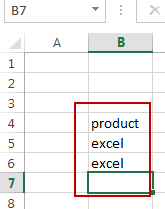# How to Copy Value of Same cell From Multiple Worksheets in Excel

This post will guide you how to copy value from same cell on multiple worksheets in your workbook in Excel. How do I List values of same cell reference from multiple worksheets with a formula in Excel. How to copy data from the same cell of multiple worksheets with VBA Macro in Excel. How to reference same cell of multiple sheets in Excel.

Assuming that you have a workbook which contain multiple worksheets and you want to extract value within the same cell (B1) from multiple worksheets (sheet1,sheet2,sheet3) into one worksheet(sheet4). How to achieve it. This post will introduce you three methods to achieve the result of copying value of same cell from multiple worksheets.

## Copying Value of Same cell From Multiple Worksheets with a Formula

To copy value of same cell from multiple worksheets in the current workbook in Excel, you can use a formula based on the INDIRECT function and the ROW function to achieve the result.

Firstly, you need to type cell reference that you want to copy into one blank cell in sheet4, such as: E1. And then you can run the following formula in another blank cell, and press Enter key in your keyboard, and drag the AutoFill Handle over other cells to apply this formula.

`=INDIRECT("'Sheet"&ROW()-1&"'!"&\$E\$1)`The INDIRECT function will refer to that cell value in E1, and the ROW function help to refer to each of source worksheet names.

## Copying Value of Same cell From Multiple Worksheets with VBA Code

You can use an Excel VBA macro to copy data within same cell across multiple worksheets in your workbook, just do the following steps:

#1 open your excel workbook and then click on “Visual Basic” command under DEVELOPER Tab, or just press “ALT+F11” shortcut.#2 then the “Visual Basic Editor” window will appear.

#3 click “Insert” ->”Module” to create a new module.#4 paste the below VBA code (get code from here)into the code window. Then clicking “Save” button.```Sub copycell()
Dim sh As Worksheet
Dim wb As Workbook
Dim DestSh As Worksheet
Dim i As Integer

With Application
.ScreenUpdating = False
.EnableEvents = False
End With

Set wb = ThisWorkbook
Set DestSh = wb.Sheets("Sheet4")

i = 4
For Each sh In ActiveWorkbook.Worksheets
If sh.Name = "Data" Then Exit Sub
sh.Range("B1").Copy
With DestSh.Range("B" & i)
.PasteSpecial xlPasteValues
.PasteSpecial xlPasteFormats
Application.CutCopyMode = False
End With
i = i + 1

Next

End Sub```

#5 back to the current worksheet, then run the above excel macro. Click Run button.#6 Let’s see the result:## Copying Value of Same cell From Multiple Worksheets with Copy & Paste Feature

There is another method to copy value of same cell from multiple sheets, and you can try to copy cell value one by one from multiple worksheets with copy & Paste feature.

You just need to press Ctrl+C keys to copy cell value and then press Ctrl+V keys to paste the value into Cell B1 in sheet4.

Video: Copying Value of Same cell From Multiple Worksheets

### Related Functions

• Excel ROW function
The Excel ROW function returns the row number of a cell reference.The ROW function is a build-in function in Microsoft Excel and it is categorized as a Lookup and Reference Function.The syntax of the ROW function is as below:= ROW ([reference])….
• Excel INDIRECT function
The Excel INDIRECT function returns the cell reference based on a text string, such as: type the text string “A2” in B1 cell, it just a text string, so you can use INDIRECT function to convert text string as cell reference….